# WFST简介

Posted by lili on

## 有穷自动机(FA)

### 确定有穷自动机(Deterministic Finite Automaton, DFA)

• Q是一个有限的状态集合
• $\Sigma$是字母表
• $\delta$是状态转移函数：$\delta: Q \times \Sigma \to Q$
• $q_0$是初始状态，$q_0 \in Q$
• F是终止状态的集合，$F \subseteq Q$

$\Sigma_i$表示字母表$\Sigma$上所有长度为i的字符串的集合。比如$\Sigma=\{a,b\}$，那么$\Sigma_1=\{a,b\}$，$\Sigma_2=\{aa, ab, ba, bb\}$，而$\Sigma_0=\{\lambda\}$。Kleene Star是定义在字母表上的一个一元操作，它是字母表上所有字符串的集合。$\Sigma^*=\Sigma_0 \cup \Sigma_1 ...$。如果$\Sigma=\{a,b\}$，那么$\Sigma^{*} = \{ \lambda, a, b, aa, ab, ba, bb, ... \}$。而Kleene Plus操作和Keene Star类似，只是它不包含空字符串。

• 点代表状态
• 边代表状态之间的跳转，边上的字符代表跳转的输入
• 我们在初始状态所在的点上画一条入边来表示它是初始状态
• 终止状态用两个圈表示

• $Q=\{a,b,c\}$
• $\Sigma=\{0,1\}$
• $q_0=a$
• $F=\{c\}$

a a b
b c a
c b c
• 表：示例DFA的转移函数图：识别ing结尾的字符串的DFA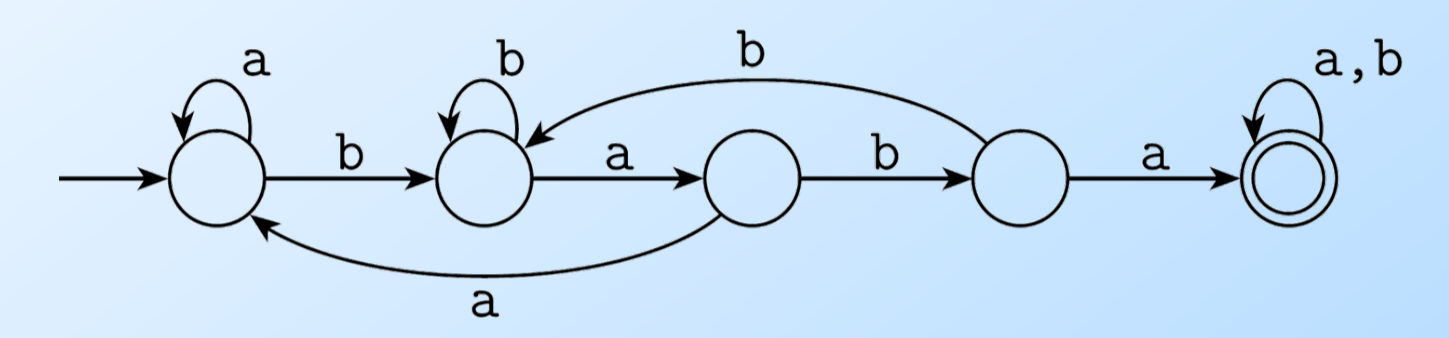图：识别包含baba的字符串的DFA

DFA的每一个状态在遇到一个字符的时候有且只有一条边跳到另一个状态(或者跳到自己)，这样的FA是确定的。如果字母表的大小是N，那么每个点出发的边一定也是N。为了简化，我们通常假设有一个“死”的状态，它不是终止状态，并且进入这个状态后遇到任何输入都是跳转到自己上。如下图所示，我们的DFA只识别”then”这个字符串，因此第一个状态只有遇到t会跳到下一个状态，其它非t的输入都会跳转到“死”状态。为了简化，我们通常可以把死状态以及跳到死状态的边去掉。这样如果DFA在某个状态遇到某个输入无法跳转(没有边可以走)就认为它进入“死”状态了。

### 非确定有穷自动机(Non-deterministic Finite Automaton/NFA)

DFA每个状态遇到任何一个输入有且仅有一个跳转(边)，我们可以对DFA进行扩展，使得一个状态遇到一个输入可以(当然不一定)有多余一条边。那这就带来一个问题：状态机执行的时候有多条边到底跳到哪个状态呢？答案是多条边都“同时”跳转，有点像状态机的“分裂”，原来只有一个状态机，现在遇到某个输入有多个跳转，一个状态机就“分裂”成多个状态机，然后到下一个输入的时候同时执行多个状态机，当然往后也可能继续“分裂”。当然某些分裂的状态机会遇到不能跳转的情况(进入死状态)，我们就认为它“死”掉了。最后字符串的字符都消费完了之后，如果至少一个分裂的状态机停止在终止状态，那么我们就认为这个状态机接受这个字符串，否则不接受。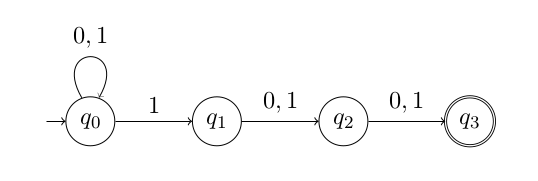图：识别倒数第3个是1的字符串的NFA

$q_0$ $q_0$ $q_1$ $q_2$ $q_3$ X(dead) X
$q_0$ $q_0$ $q_1$ $q_2$ $q_3$(accepted)
$q_0$ $q_0$ $q_1$
$q_1$

### 通过子集构造把NFA转换成DFA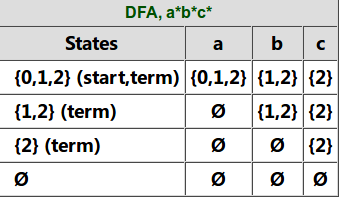图：通过子集构造把NFA转换成DFA

## 加权有限状态转换器(WFST)

### 基本概念

#### 加权的接受器(Weighted Acceptors)

WFSA在FSA的基础上输出一个权重，下图是在语音识别中使用WFSA的几个例子。下图(a)是语言模型的例子，下图(b)是发音词典，而下图(c)是一个音子的HMM，每个音子都三状态从左到右的HMM，因此它可以接受”d1+ d2+ d3+”，+表示出现一次或者多次。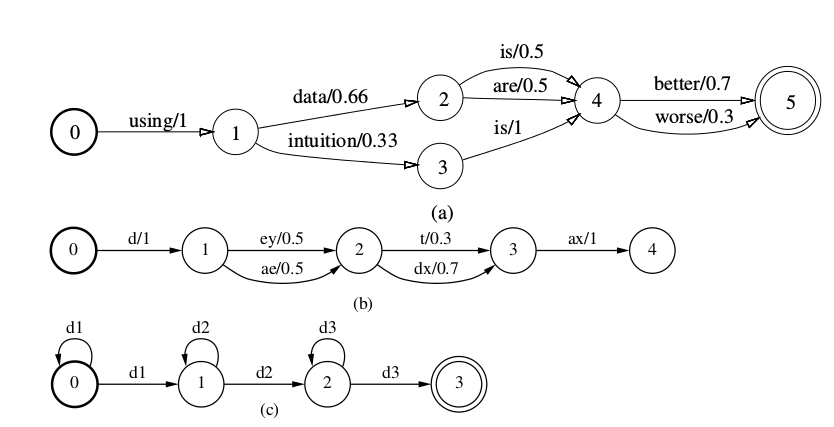图：WFSA在语音识别中的例子

#### 加权的转换器(Weighted Transducers)图：WFST在语音识别中的例子

#### 确定化(Determinization)操作图：WFSA Determinization示例

#### 最小化(Minimization)操作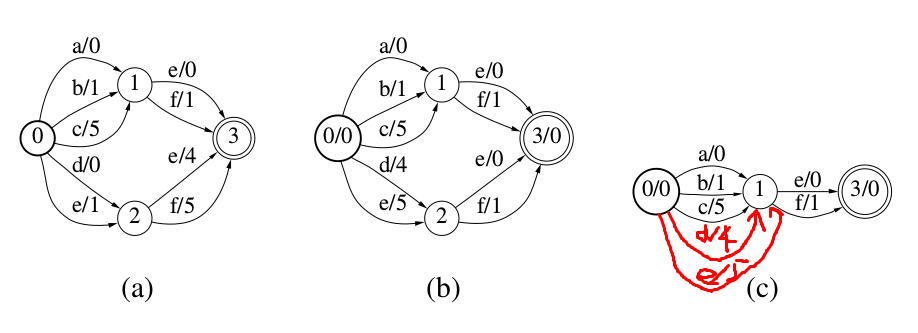图：WFSA Minimization示例

weight pushing是一种特殊的reweighting，我们下面用tropical 半环为例来说明reweighting，其它半环是类似的。关于半环(semi-ring)后面会有介绍，读者暂时不用管它。

$w[t] \leftarrow w[t] + (V(n[t]) - V(p[t]))$

$i \overset{t_1}{\rightarrow} v_1 \overset{t_2}{\rightarrow} v_2 \overset{t_3}{\rightarrow} f$

$\rho[i] + w[t_1] + w[t_2] + w[t_3] + \rho[f]$

$\begin{split} & \rho[i] + w[t_1] +(V(v_1)-V(i) + w[t_2] +(V(v_2)-V(v_1)) + \\ & \;\;\;\;\;\;\;\;\;\; w[t_3]+(V(f)-V(v_2)) + \rho[f] +(V(i) -V(f)) \\ & = \rho[i] + w[t_1] + w[t_2] + w[t_3] + \rho[f] +[V(v_1)-V(i)+V(v_2)-V(v_1)+V(f)-V(v_2)+V(i)-V(f)] \\ & = \rho[i] + w[t_1] + w[t_2] + w[t_3] + \rho[f] \end{split}$

#### 算法实现

WFST的Composition、Determinization和Minimization的具体算法这里就不详细介绍，有兴趣的读者可以阅读Speech Recognition with Weighted Finite State Transducers等相关论文。我们通常也不需要自己实现这些算法，OpenFst等开源库提供了高效的实现，而语音识别工具Kaldi更是对OpenFst进行了封装，使得它更加适合语音识别的任务。

### 半环和WFST的形式化定义

• 加法满足交换律和结合律

也就是$\forall x,y\;$，有：

$x \bigoplus y = y \bigoplus x$ $(x \bigoplus y) \bigoplus z = x \bigoplus (y \bigoplus z)$
• 零元加任何元素不变

$\bar{0} \bigoplus x =x \bigoplus \bar{0} =x$
• 乘法满足结合律和对加法的分配率

$(x \bigotimes y) \bigotimes z = x \bigotimes (y \bigotimes z)$ $(x \bigoplus y) \bigotimes z= x \bigotimes z \bigoplus y \bigotimes z$ $x \bigotimes (y \bigoplus z)= x \bigotimes y \bigoplus x \bigotimes z$
• 零元乘以数也不变

$\bar{1} \bigotimes x=x \bigotimes \bar{1}=x$
• 零元乘以任何数等于零(吸收律)

$\bar{0} \bigotimes x =x \bigotimes \bar{0} = \bar{0}$

• 加法满足交换律和结合律
$\begin{split} & x \bigoplus y = min(x,y)=min(y,x)=y \bigoplus x \\ & (x \bigoplus y) \bigoplus z=min(min(x,y),z)=min(x,min(y,z))=x \bigoplus (y \bigoplus z) \end{split}$
• 零元加任何元素不变
$\bar{0} \bigoplus x = min(+\infty,x)=x$
• 乘法满足结合律和对加法的分配率
$\begin{split} & (x \bigotimes y) \bigotimes z = (x+y)+z=x+(y+z)=x \bigotimes (y \bigotimes z) \\ & (x \bigoplus y) \bigotimes z = min(x,y) + z = min(x+z, y+z)=(x \bigotimes z) \bigoplus (y \bigotimes z) \end{split}$
• 零元乘以数也不变
$\bar{1} \bigotimes x=0 + x=x$
• 零元乘以任何数等于零(吸收律)
$\bar{0} \bigotimes x =+\infty + x =+\infty = \bar{0}$

• $\mathcal{A}$是一个有限的输入字母表
• $\mathcal{B}$是一个有限的输出字母表
• Q是一个有限的状态集合
• $I \subseteq Q$是初始状态集合
• $F \subseteq Q$是终止状态集合
• $E \subseteq Q \times (\mathcal{A} \cup {\epsilon}) \times (\mathcal{B} \cup {\epsilon}) \times \mathbb{K} \times Q$
• $\lambda : I \rightarrow \mathbb{K}$，给初始状态一个weight
• $\rho: F \rightarrow \mathbb{K}$，给终止状态一个weight

$T_1 \circ T_2(x, y) = \underset{z \in \mathcal{B}^*}{\bigoplus}T_1(x,z) \bigotimes T_2(z,y)$

## WFST在语音识别中的应用

### G

G表示语言模型。它是一个WFSA，它的输入是一个词序列，然后G可以判断这个词序列是否符合语法以及它的概率。对于固定的文法来说，它只能识别符合文法的句子；而对于N-Gram来说，所有的句子都是可能的，只不过概率有高有低。

### L

L是发音词典。它的输入(上下文无关的)因子序列，输出是词序列。比如上面文法对应的发音词典：

bill b ih l
jill jh ih l
jim jh ih m
red r eh d
...


$0-(b:bill)->1，1-(ih:\epsilon)->2，2-(l:\epsilon)->3$

$3-(\#1:\epsilon)->4$

read r eh d #0
red r eh d #1


### C

C 是把上下文相关的因子序列转换成上下文无关的因子序列的WFST。声学模型的输出是上下文相关的因子序列，而L要求的输入是上下文无关的因子序列，因此我们需要C来把上下文相关的因子序列转换成上下文无关的。

"k,ae" -t-> ae/k_t
"k,ae" -d-> ae/k_d
....


### H

H是把声学观察序列变成上下文相关因子序列的WFST。这个WFST代表声学模型，它的输入是观察序列，输出是上下文相关因子序列及其概率。

$N=\pi_\epsilon(min(det(\tilde{H} \circ det(\tilde{C} \circ det(\tilde{L} \circ G)))))$